# Estimating Temperature and Precipitation at the Last Glacial Maximum

William W. Hargrove and Jonathan Adams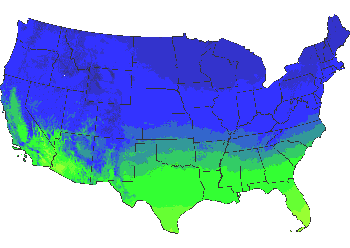## Estimating Valdai Temperatures

Estimating temperatures during the Valdai last glacial maximum was a three-step process: interpolating a continuous delta temperature surface, obtaining a continuous map of current mean annual temperature, and subtracting the delta surface from the current map to produce a Valdai mean temperature map.

### Interpolating the Temperature Differences Grid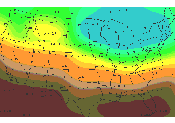This is the map of the final interpolated delta temperature grid. An initial delta temperature grid was obtained from Frenzel et. al (1992), showing the differential between current temperatures and those estimated during the Valdai glaciation. This discontinuous delta temperature grid was interpolated using a regularized spline with tension and smoothing into a continuous temperature differential surface. The tension parameter was set to 40 and smoothing was set to 2, with zmult=10. Resolution of the temperature differential map is 10 kilometers, and units are delta degrees Celsius.

### Current Mean Annual Temperatures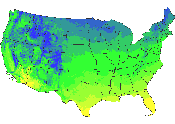A national map of mean annual temperature was interpolated from over 4500 meterological stations. Initially, a map of relative humidity was interpolated. This map was used to generate a spatially-customized adiabatic lapse rate, which is dependent on moisture content of the air. Using the customized lapse rate, station temperatures were "adjusted" to what they would have been at Mean Sea Level (MSL). The interpolation was performed on the MSL temperatures.

After interpolating, the same custom lapse rate, along with a Digital Elevation Model from the North American portion of the GTOPO30 global elevation data set was used to "re-adjust" the temperatures in each cell back to the appropriate elevation. Resolution of the current mean annual temperature map is one square kilometer, and units are tenths of degrees Farenheit.

### Valdai Mean Annual Temperatures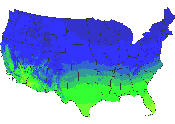The national map of estimated mean annual Valdai temperatures was produced by subtracting the interpolated delta temperature surface from the current temperature map. Resolution of the Valdai mean temperature map is one square kilometer, and units are tenths of degrees Farenheit.

## Estimating Valdai Precipitation

Estimating precipitation during the Valdai last glacial maximum required a similar three-step process as did temperature.

### Interpolating the Precipitation Differences Grid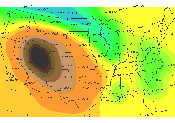This is the final interpolated delta precipitation grid. An initial delta precipitation grid was obtained from Frenzel et. al (1992), showing the differential between current precipitation and those estimated during the Valdai glaciation. This discontinuous delta precipitation grid was interpolated using a regularized spline with tension and smoothing into a continuous precipitation differential surface. The tension parameter was set to 40 and smoothing was set to 2. Resolution of the precipitation differential map is 10 kilometers, and units are delta mm precipitation.

### Current Mean Annual Precipitation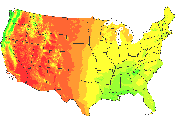Orographically -corrected mean annual precipitation from Chris Daly's PRISM model gives mean annual rainfall equivalent in mm at 4x4 km resolution which has been corrected for elevation effects.

### Valdai Mean Annual Precipitation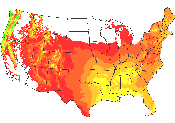The national map of estimated mean annual Valdai precipitation was produced by subtracting the interpolated delta precipitation surface from the current precipitation map. Negative precipitation resulting from the subtraction was reset to zero. Resolution of the Valdai mean precipitation map is four square kilometers, and units are mm rainfall equivalent.

## Literature Cited

Frenzel B., Pecsi B & Velichko A.A. (1992) (Ed.s). Atlas of Palaeoclimates & Palaeoenvironments of the Northern Hemisphere. INQUA/Hungarian Academy of Sciences. Budapest.